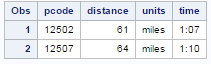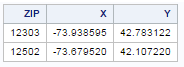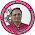# Calculating Driving Distance with SAS

This tutorial demonstrates how to calculate driving distance with SAS. Calculating travelling distance between two locations is an important step of Geo Spatial Analysis. Today geo analytics or network analysis is very much in demand. The main idea is to find the shortest path based on existing road networks, or calculate actual travel time or estimate the fair trip based on distance. For example, you hire a private cab (taxi) through Uber App. Prior of booking a cab, you can estimate the fair trip. Open source softwares such as R and Python supports various packages to solve this kind of problem. SAS also has a couple of in-built functions to calculate distance between two points.

Ways to measure traveling distance

There are multiple ways to compute geographical distance. Some of the methods are listed below -
1. Driving Distance via Google Map
2. Straight Line Distance such as Euclidean
3. Manhattan (Taxi cab distance)
4. Vincenty distance formula
5. Haversine distance formula

Which method is more suitable
1. Driving distance via Google map is most accurate when you need to compute the real driving distance which includes waiting time at traffic signals or real-time traffic in some places between two locations.
2. Straight line distance (Euclidean) is more suitable when you need to calculate the distance between two trees. Or if you are traveling via plane as it ignores earth curvature.
3. Manhattan Distance (Taxi Cab) is more suitable when you are travelling through cab/taxi. It is better measure when you need to determine the traveling distance between customer's location and office location.
4. Vincenty or Haversine Distance are calculated based on latitude and longitude of the zip code or postcode. Vincenty is generally more accurate than Haversine method. Vincenty distance between two points is accurate to within 0.5 mm distance.

SAS : Driving Distance via Google Map

In SAS, there is no in-built function to connect to google map and extract driving distance. To workaround this problem, we need to connect to google map via weblink. Check out the link below - https://www.google.com/maps/dir/110051/110053?force=lite

In this link, 110051 is a starting point and 110053 is a destination point.

SAS Macro to extract driving distance and time
*Starting point;
%let p1=12303;

* Destination Points (postcodes);
data postcodes;
input pcode @@;
datalines;
12502 12507
;

proc datasets lib=work nolist;
delete driving_distance;
quit;

data _null_;
call symputx('ncodes',obs);
stop;
set postcodes nobs=obs;
run;

* Macro to connect to Google Map;
%macro driving_distance;
%do j=1 %to &ncodes;
data _null_;
nrec = &j;
set postcodes point=nrec;
call symputx('p2',put(pcode,z5.));
stop;
run;

%put &p1 &p2;
filename z temp;

data _null_;
infile x recfm=f lrecl=1 end=eof;
file z recfm=f lrecl=1;
input @1 x \$char1.;
put @1 x \$char1.;
if eof;
call symputx('filesize',_n_);
run;

* drive time as a numeric variable;
data temp;
retain pcode &p2;
infile z recfm=f lrecl=&filesize. eof=done;
input @ 'miles' +(-15) @ '"' distance :comma12. text \$30.;
units    = scan(text,1,'"');
text     = scan(text,3,'"');

* convert times to seconds;
select;
* combine days and hours;
when (find(text,'d') ne 0)  time = sum(86400*input(scan(text,1,' '),best.),
3600*input(scan(text,3,' '),best.));
* combine hours and minutes;
when (find(text,'h') ne 0)  time = sum(3600*input(scan(text,1,' '),best.),
60*input(scan(text,3,' '),best.));
* just minutes;
otherwise                   time = 60*input(scan(text,1,' '),best.);
end;

output;
keep pcode distance units time;
stop;
done:
output;
run;

filename x clear;
filename z clear;

proc append base=driving_distance data=temp;
run;
%end;
%mend;

* use the macro;
%driving_distance;

proc print data=driving_distance;
format pcode z5. distance comma6. time time6.;
run;
The output is shown in the image below -SAS Driving Distance and Time
Straight Line Distance / Euclidean Distance

Euclidean distance measure is one of the most popular method to calculate distance between two points. It is also used in cluster analysis to see the similarity between two observations.
Euclidean = √( x2 - x1)² + (y2 - y1)²
In the geographical points, x and y coordinates are easting and northing. Easting refers to the eastward measure distance (x coordinate) and Northing refers to the northward measure distance (y coordinate)

For zip code - 12502,  x and y coordinates are listed below -
x - 610435  y - 4660250
For zip code - 12303, x and y coordinates are as follows -
x - 589594  y - 4732566
data _null_;
x1 = 610435;
y1 = 4660250;
x2 = 589594;
y2 = 4732566;
euclidean_dist =  round(sqrt(( x2 - x1)**2 + (y2 - y1)**2)/1609,0.1);
put euclidean_dist =;
run;
Result - 46.8 miles

Since it returns the distance in metres, we need to divide it by 1609. 1609 metres is equal to 1 mile. If you want to measure distance in km, you need to divide it by 1000. The resulted value 46.8 is far below than actual distance of 61 miles. Since Euclidean distance ignores earth curvature, it's not a good approximation of actual driving distance.

Manhattan Distance

It is a good approximation of actual driving distance. It is also called taxi cab distance. It is useful when we need to compute the road distance between two geographical points.
Manhattan  = (abs(x2 - x1) + abs(y2 - y1)
data _null_;
x1 = 610435;
y1 = 4660250;
x2 = 589594;
y2 = 4732566;
Manhattan_dist =  round((abs(x2 - x1) + abs(y2 - y1))/1609,0.1);
put Manhattan_dist =;
run;
Result - 57.9 miles

Vincenty or Haversine Distance

SAS has two inbuilt functions - ZIPCITYDISTANCE and GEODIST which are based on Vincenty distance method to compute geographical distance.

The ZIPCITYDISTANCE function uses latitude and longitude from the SASHELP.ZIPCODE data set and computes the distance between zip code centroids for any two zip codes specified by the user.
proc print data = SASHELP.ZIPCODE (where = (ZIP IN(12502, 12303))) noobs;
var zip x y;
run;LAT / LON
In this case, Y refers to latitude and X refers to longitude. In ZIPCITYDISTANCE function, you just need to specify zip codes.
data _null_;
zip_dist = zipcitydistance(12502, 12303);
put zip_dist =;
run;
Result : 48.5 miles

Note : The ZIPCITYDISTANCE function works only for US zipcodes.

The GEODIST functions works for all the countries. In the function, you need to specify latitude and longitude of zip codes.
data _null_;
long1 = -73.679520;
lat1 = 42.107220;
long2 = -73.938595;
lat2 = 42.783122;
zip_dist = geodist(lat1, long1, lat2, long2, 'M');
put zip_dist =;
run;
It returns 48.5 miles (same as ZIPCITYDISTANCE) as both the functions are based on the same algorithm i.e. vincenty distance.

Haversine Distance
data _null_;
long1 = -73.679520;
lat1 = 42.107220;
long2 = -73.938595;
lat2 = 42.783122;
conversion = constant('pi')/180;
lat1  = lat1  * conversion;
long1 = long1 * conversion;
lat2  = lat2  * conversion;
long2 = long2 * conversion;
zip_dist = 3949.99 * arcos(sin(lat1) * sin(lat2) + cos(lat1) *
cos(lat2) * cos(long2 - long1));

put zip_dist =;
run;
Result : 48.4 miles

Comparison of Distance Methods

Google Map Euclidean Manhattan Vincenty Haversine
61 46.8 57.9 48.5 48.4
Calculating driving distance via Google Map is the most precise method. But it is not efficient in terms of speed as it takes time to connect and pull values from map. Sometimes windows firewall blocks program to connect to google map. In that case, we should use manhattan distance as it stands out as a best estimation of actual driving distance.Related PostsDeepanshu founded ListenData with a simple objective - Make analytics easy to understand and follow. He has over 8 years of experience in data science. During his tenure, he has worked with global clients in various domains like Banking, Insurance, Telecom and Human Resource.

3 Responses to "Calculating Driving Distance with SAS"
1.2.Rather than utilizing a guide, a ruler distance calculator UK and the key that clarifies the size of the guide, you can basically include the two urban areas and discover the course to distance between urban communities. This significantly disentangles the procedure.

3.Great post, beautiful weblog with great informational content. This is a really interesting and informative content. Jubilee Car Insurance - united car insurance

Next → ← Prev
Love this Post? Spread the Word!
Share